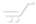# The if operator

The "if" operator.
The basic syntax of the "if" operator has four elements that must appear in the following order:
1. The "if" keyword.
2. formula.
3. The "then" key word.
4. formula.
For example:
If 4>5 then "bigger"

Usage in formula
This operator is no different than the other operators in the sense that it can be combined with other formula terms and operators to form more complex formulas. For example:
(if 4<5 then 4)+ 4.

The "else" clause
The "if" operator can have the optional "else" clause. This clause has two elements that must be in the exact following order:
1. The "else" keyword.
2. formula.
For example:
if 4<5 then "bigger" else "it is smaller"

Functionality
When an if operator is evaluated, the formula following the "if" keyword is evaluated first and converted to boolean, if needed. If this value is the "true" boolean value, then the value of the entire operator is the value of the formula following the "then" keyword.

Otherwise, the value of the this operator is the value of the formula in the "else" clause, if present, or null value if there is no else clause.